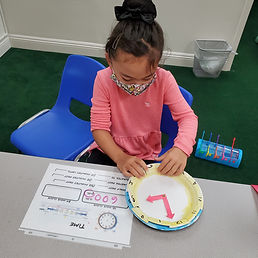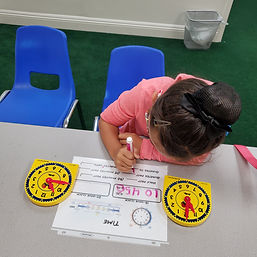## Ms. Raejean

### Target 1​

###### Lesson Type:

New

Algebra

:

Variable

Solve equations that use variables to represent unknown numbers.

###### 1:

Identify the unknown information in problems.

3rd

###### Vocabulary:

Multi-step, Equation, Equal, Unknown Numbers, Variable, Inverse Operation, Regrouping

Activities:

Students solved multi-step equations. Problems were solved left to right. Then students solved for the unknown number using the inverse operation. Students practiced regrouping with base-ten blocks.

Ex. 10 + p + 18 - 12 = 34

Step 1: 10 + 18 = 28

Step 2: 28 - 12 = 16

Step 3: 16 + p = 34, so 34 - 16 = p.### Home Exploration

###### Guiding Questions:## Absent Students:

### Target 2

:

###### 1:

Learn the meaning of time expressions such as half-past, quarter-past, and quarter-to.

###### 2:

Understand and use 24-hour notation (military time).

###### 3:

Link digital, analogy, a.m., p.m., and military time.

3rd

###### Vocabulary:

12-hour clock, 24-hour clock, Half past, Quarter to, Quarter past, Digital, Analogue, A.M., P.M., Military time

Activities:

Students watched a video comparing 12-hour and 24-hour clocks. Then students made 24-hour clocks. The top plate represents the first 12 hours (1-12), and the bottom plate represents the next 12 hours (13-24 or 00).

Students made the time on the 24-hour clock, then wrote the time as it would appear on a digital clock and in military time. Ex. 6:00 p.m. is 1800.

Students also practiced using the phrases half past, quarter past, and quarter to. Students made the time on the clock and completed the appropriate phrase. Ex. 10:45 p.m. is a quarter to 11.

We will continue to practice using military time and using the phrases.### Home Exploration

###### Guiding Questions:### Target 3

:

###### Vocabulary:

Activities:### Home Exploration